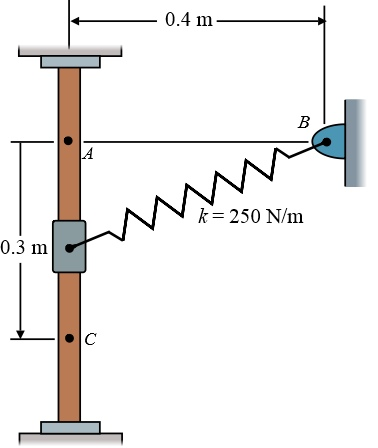# The vertical guide is smooth and the 4-Kg collar is released from rest at A. Part A Determine...

## Question:

The vertical guide is smooth and the 4-Kg collar is released from rest at A.

Part A

Determine the speed of the collar when it is at position C. The spring has an unstretched length of 350 mm. (Figure below)

Express your answer with the appropriate units.## Spring Energy:

The potential energy in the spring in the form of its un stretched length due to which deflection of spring occurs is known as spring energy. It depends on the spring stiffness and the deflection in the spring.

## Answer and Explanation:

Given data:

• Mass of collar is: {eq}m = 4\;{\rm{kg}} {/eq}
• Un stretched length of the spring is: {eq}x = 350\;{\rm{mm}} = 0.35\;{\rm{m}} {/eq}
• Length of AB is: {eq}AB = 0.4\;{\rm{m}} {/eq}
• Length of AC is: {eq}AC = 0.3\;{\rm{m}} {/eq}
• Spring stiffness is: {eq}k = 250\;{\rm{N/m}} {/eq}

Expression for the stretched length of the spring at point A.

{eq}{x_A} = AB - x {/eq}

Substitute the values in the above expression.

{eq}\begin{align*} {x_A} &= 0.4\;{\rm{m}} - 0.35\;{\rm{m}}\\ {x_A} &= 0.05\;{\rm{m}} \end{align*} {/eq}

Formula for the spring energy at point A.

{eq}S.{E_1} = \dfrac{1}{2}k{x_A}^2 {/eq}

Substitute the values in the above expression.

{eq}\begin{align*} S.{E_1} &= \dfrac{1}{2}\left( {250\;{\rm{N/m}} \times \dfrac{{1\;{\rm{kg}} \cdot {\rm{m}}/{{\rm{s}}^2}}}{{1\;{\rm{N}}}}} \right){\left( {0.05\;{\rm{m}}} \right)^2}\\ &= 0.3125\;{\rm{kg}} \cdot {{\rm{m}}^2}/{{\rm{s}}^2} \times \dfrac{{1\;{\rm{J}}}}{{1\;{\rm{kg}} \cdot {{\rm{m}}^2}/{{\rm{s}}^2}}}\\ S.{E_1} &= 0.3125\;{\rm{J}} \end{align*} {/eq}

Formula for the initial potential energy.

{eq}P.{E_1} = mg\left( {AC} \right) {/eq}

Here, the acceleration due to gravity is {eq}g = 9.81\;{\rm{m/}}{{\rm{s}}^2}. {/eq}

Substitute the values in the above expression.

{eq}\begin{align*} P.{E_1} &= \left( {4\;{\rm{kg}}} \right)\left( {9.81\;{\rm{m/}}{{\rm{s}}^2}} \right)\left( {0.3\;{\rm{m}}} \right)\\ P.{E_1} &= 11.72\;{\rm{kg}} \cdot {{\rm{m}}^2}/{{\rm{s}}^2} \times \dfrac{{1\;{\rm{J}}}}{{1\;{\rm{kg}} \cdot {{\rm{m}}^2}/{{\rm{s}}^2}}}\\ P.E &= 11.72\;{\rm{J}} \end{align*} {/eq}

Formula to determine the length of BC.

{eq}BC = \sqrt {A{B^2} + A{C^2}} {/eq}

Substitute the values in the above expression.

{eq}\begin{align*} BC &= \sqrt {{{\left( {0.4\;{\rm{m}}} \right)}^2} + {{\left( {0.3\;{\rm{m}}} \right)}^2}} \\ BC &= \sqrt {0.25\;{{\rm{m}}^2}} \\ BC &= 0.5\;{\rm{m}} \end{align*} {/eq}

Expression for the stretched length of the spring at point C.

{eq}{x_C} = BC - x {/eq}

Substitute the values in the above expression.

{eq}\begin{align*} {x_C} &= 0.5\;{\rm{m}} - 0.35\;{\rm{m}}\\ {x_C} &= 0.15\;{\rm{m}} \end{align*} {/eq}

Formula for the spring energy at point C.

{eq}S.{E_2} = \dfrac{1}{2}k{x_C}^2 {/eq}

Substitute the values in the above expression.

{eq}\begin{align*} S.{E_2} =& \dfrac{1}{2}\left( {250\;{\rm{N/m}} \times \dfrac{{1\;{\rm{kg}} \cdot {\rm{m}}/{{\rm{s}}^2}}}{{1\;{\rm{N}}}}} \right){\left( {0.15\;{\rm{m}}} \right)^2}\\ &= 2.8125\;{\rm{kg}} \cdot {{\rm{m}}^2}/{{\rm{s}}^2} \times \dfrac{{1\;{\rm{J}}}}{{1\;{\rm{kg}} \cdot {{\rm{m}}^2}/{{\rm{s}}^2}}}\\ S.{E_2} &= 2.8125\;{\rm{J}} \end{align*} {/eq}

Formula for the kinetic energy at point C.

{eq}K.{E_2} = \dfrac{1}{2}m{v^2} {/eq}

Here, the velocity at point C is {eq}v. {/eq}

Substitute the values in the above expression.

{eq}\begin{align*} K.{E_2} &= \dfrac{1}{2}\left( 4 \right){v^2}\\ K.{E_2} &= 2{v^2} \end{align*} {/eq}

Consider the law f conservation of energy between point A and point C.

{eq}K.{E_1} + S.{E_1} + P.{E_1} = K.{E_2} + S.{E_2} + P.{E_2} {/eq}

Here, the kinetic energy at point A is {eq}K.{E_1} = 0 {/eq} as at point A velocity of collar is zero and potential energy at point C is {eq}P.{E_2} = 0 {/eq} as the height is zero.

Substitute the values in the above expression.

{eq}\begin{align*} 0 + 0.3125 + 11.72 = 2{v^2} + 2.8125 + 0\\ 12.0325 &= 2{v^2} + 2.8125\\ 2{v^2} &= 9.22\\ {v^2} &= 4.61\\ v &= 2.417\;{\rm{m/s}} \end{align*} {/eq}

Thus, speed of the collar when it is at position C is {eq}2.417\;{\rm{m/s}}. {/eq}